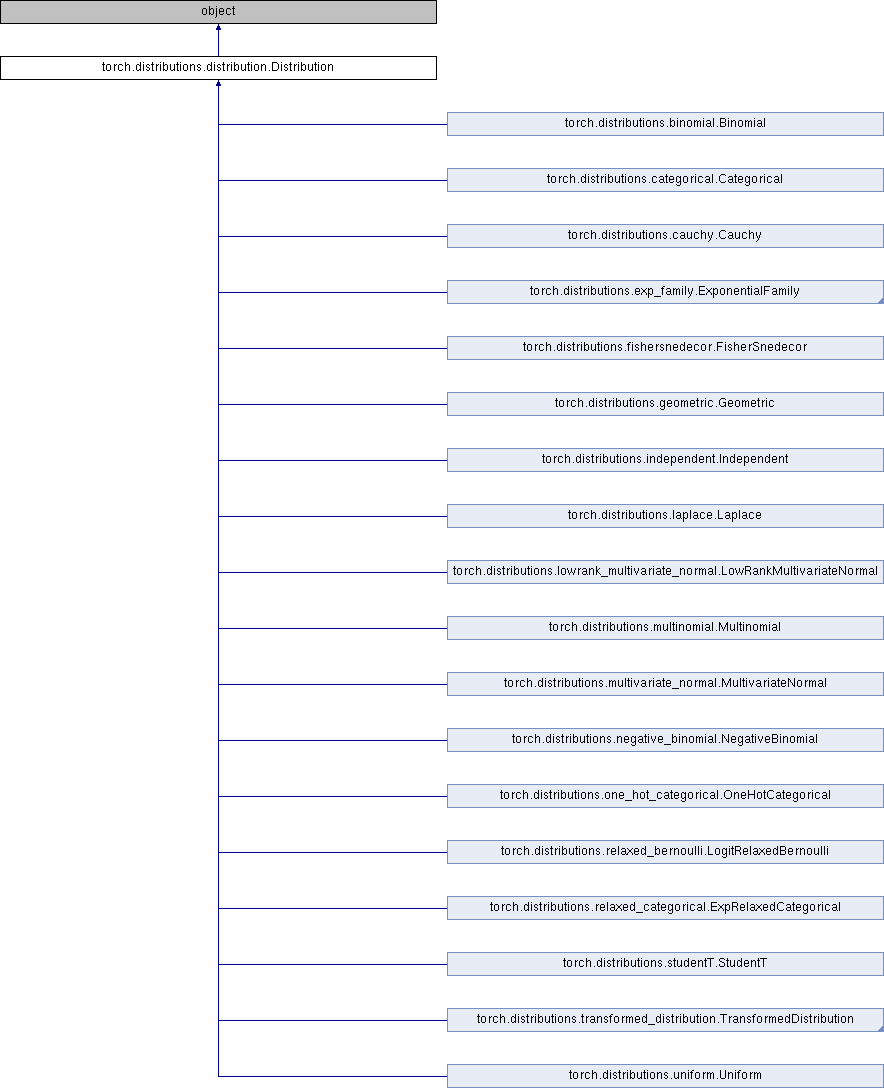Caffe2 - Python API A deep learning, cross platform ML framework
torch.distributions.distribution.Distribution Class Reference
Inheritance diagram for torch.distributions.distribution.Distribution:## Public Member Functions

def __init__ (self, batch_shape=torch.Size(), event_shape=torch.Size(), validate_args=None)

def expand (self, batch_shape, _instance=None)

def batch_shape (self)

def event_shape (self)

def arg_constraints (self)

def support (self)

def mean (self)

def variance (self)

def stddev (self)

def sample (self, sample_shape=torch.Size())

def rsample (self, sample_shape=torch.Size())

def sample_n (self, n)

def log_prob (self, value)

def cdf (self, value)

def icdf (self, value)

def enumerate_support (self, expand=True)

def entropy (self)

def perplexity (self)

def __repr__ (self)

## Static Public Member Functions

def set_default_validate_args (value)

## Static Public Attributes

has_rsample

has_enumerate_support

support

arg_constraints

## Detailed Description

Definition at line 7 of file distribution.py.

## Member Function Documentation

 def torch.distributions.distribution.Distribution.arg_constraints ( self )
```Returns a dictionary from argument names to
:class:`~torch.distributions.constraints.Constraint` objects that
should be satisfied by each argument of this distribution. Args that
are not tensors need not appear in this dict.
```

Definition at line 75 of file distribution.py.

 def torch.distributions.distribution.Distribution.batch_shape ( self )
```Returns the shape over which parameters are batched.
```

Definition at line 61 of file distribution.py.

 def torch.distributions.distribution.Distribution.cdf ( self, value )
```Returns the cumulative density/mass function evaluated at
`value`.

Args:
value (Tensor):
```

Definition at line 147 of file distribution.py.

 def torch.distributions.distribution.Distribution.entropy ( self )
```Returns entropy of distribution, batched over batch_shape.

Returns:
Tensor of shape batch_shape.
```

Definition at line 191 of file distribution.py.

 def torch.distributions.distribution.Distribution.enumerate_support ( self, expand = `True` )
```Returns tensor containing all values supported by a discrete
distribution. The result will enumerate over dimension 0, so the shape
of the result will be `(cardinality,) + batch_shape + event_shape`
(where `event_shape = ()` for univariate distributions).

Note that this enumerates over all batched tensors in lock-step
`[[0, 0], [1, 1], ...]`. With `expand=False`, enumeration happens
along dim 0, but with the remaining batch dimensions being
singleton dimensions, `[, , ..`.

To iterate over the full Cartesian product use
`itertools.product(m.enumerate_support())`.

Args:
expand (bool): whether to expand the support over the
batch dims to match the distribution's `batch_shape`.

Returns:
Tensor iterating over dimension 0.
```

Definition at line 167 of file distribution.py.

 def torch.distributions.distribution.Distribution.event_shape ( self )
```Returns the shape of a single sample (without batching).
```

Definition at line 68 of file distribution.py.

 def torch.distributions.distribution.Distribution.expand ( self, batch_shape, _instance = `None` )
```Returns a new distribution instance (or populates an existing instance
provided by a derived class) with batch dimensions expanded to
`batch_shape`. This method calls :class:`~torch.Tensor.expand` on
the distribution's parameters. As such, this does not allocate new
memory for the expanded distribution instance. Additionally,
this does not repeat any args checking or parameter broadcasting in
`__init__.py`, when an instance is first created.

Args:
batch_shape (torch.Size): the desired expanded size.
_instance: new instance provided by subclasses that
need to override `.expand`.

Returns:
New distribution instance with batch dimensions expanded to
`batch_size`.
```

Definition at line 39 of file distribution.py.

 def torch.distributions.distribution.Distribution.icdf ( self, value )
```Returns the inverse cumulative density/mass function evaluated at
`value`.

Args:
value (Tensor):
```

Definition at line 157 of file distribution.py.

 def torch.distributions.distribution.Distribution.log_prob ( self, value )
```Returns the log of the probability density/mass function evaluated at
`value`.

Args:
value (Tensor):
```

Definition at line 137 of file distribution.py.

 def torch.distributions.distribution.Distribution.mean ( self )
```Returns the mean of the distribution.
```

Definition at line 93 of file distribution.py.

 def torch.distributions.distribution.Distribution.perplexity ( self )
```Returns perplexity of distribution, batched over batch_shape.

Returns:
Tensor of shape batch_shape.
```

Definition at line 200 of file distribution.py.

 def torch.distributions.distribution.Distribution.rsample ( self, sample_shape = `torch.Size()` )
```Generates a sample_shape shaped reparameterized sample or sample_shape
shaped batch of reparameterized samples if the distribution parameters
are batched.
```

Definition at line 121 of file distribution.py.

 def torch.distributions.distribution.Distribution.sample ( self, sample_shape = `torch.Size()` )
```Generates a sample_shape shaped sample or sample_shape shaped batch of
samples if the distribution parameters are batched.
```

Definition at line 113 of file distribution.py.

 def torch.distributions.distribution.Distribution.sample_n ( self, n )
```Generates n samples or n batches of samples if the distribution
parameters are batched.
```

Definition at line 129 of file distribution.py.

 def torch.distributions.distribution.Distribution.stddev ( self )
```Returns the standard deviation of the distribution.
```

Definition at line 107 of file distribution.py.

 def torch.distributions.distribution.Distribution.support ( self )
```Returns a :class:`~torch.distributions.constraints.Constraint` object
representing this distribution's support.
```

Definition at line 85 of file distribution.py.

 def torch.distributions.distribution.Distribution.variance ( self )
```Returns the variance of the distribution.
```

Definition at line 100 of file distribution.py.

The documentation for this class was generated from the following file: